# Chapter 3 Making Maps in R

Learning Objectives

• plot an `sf` object
• create a choropleth map with `ggplot`
• add a basemap with `ggmap`
• use `RColorBrewer` to improve legend colors
• use `classInt`to improve legend breaks
• create a choropleth map with `tmap`
• create an interactive map with `leaflet`
• customize a `leaflet` map with popups and layer controls

In the preceding examples we have used the base `plot` command to take a quick look at our spatial objects.

In this section we will explore several alternatives to map spatial data with R. For more packages see the “Visualisation” section of the CRAN Task View.

Mapping packages are in the process of keeping up with the development of the new `sf` package, so they typicall accept both `sp` and `sf` objects. However, there are a few exceptions.

Of the packages shown here `spplot()`, which is part of the good old `sp` package, only takes `sp` objects. The development version of `ggplot2` can take `sf` objects, though `ggmap` seems to still have issues with `sf`. Both `tmap` and `leaflet` can also handle both `sp` and `sf` objects.

## 3.1 Plotting simple features (`sf`) with `plot`

As we have already briefly seen, the `sf` package extends the base `plot` command, so it can be used on `sf` objects. If used without any arguments it will plot all the attributes.

``````philly_crimes_sf <-  st_read("data/PhillyCrimerate/", quiet = TRUE)
plot(philly_crimes_sf)``````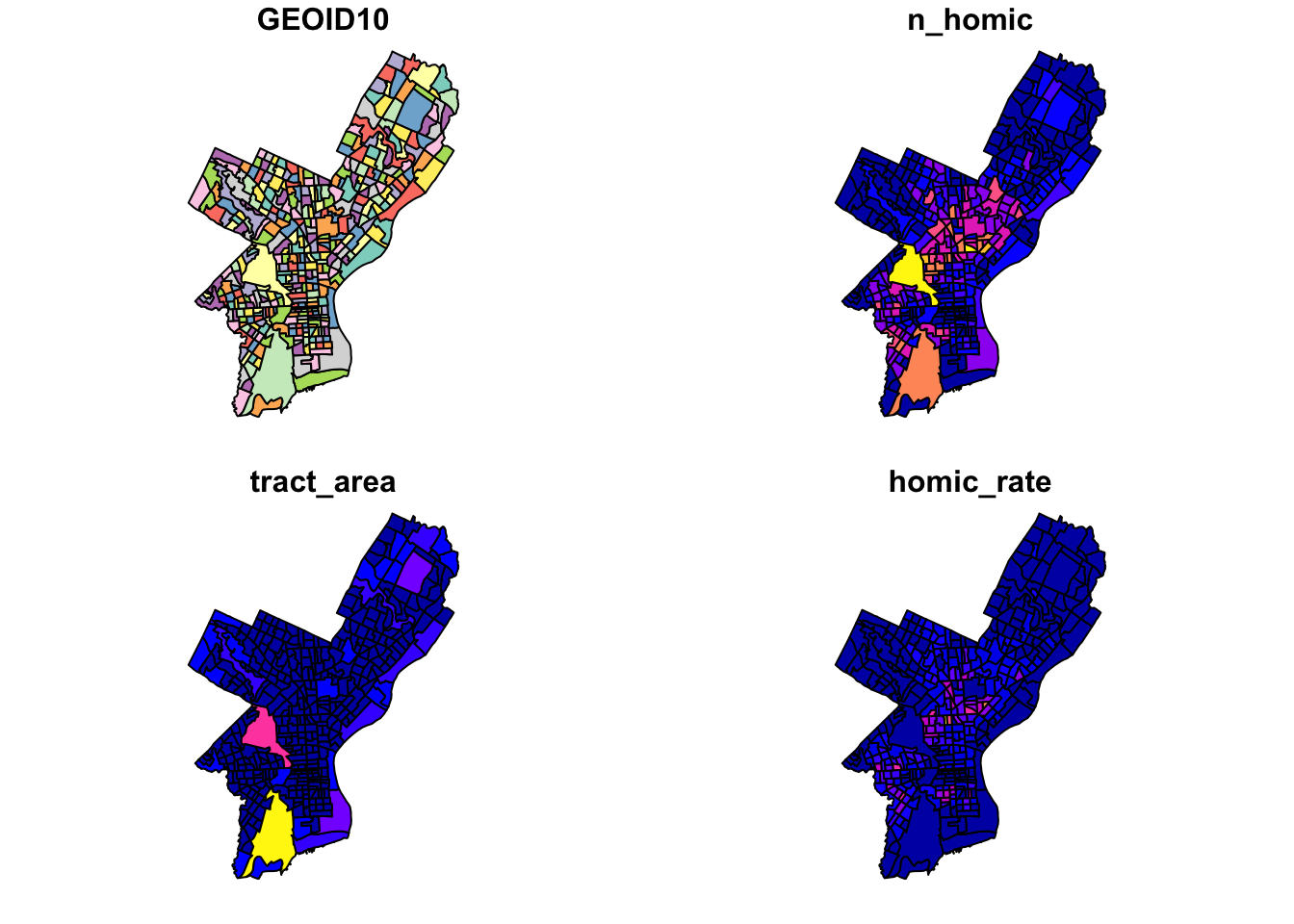To plot a single attribute we need to provide an object of class `sf`, like so:

``plot(philly_crimes_sf\$homic_rate) # this is a numeric vector!``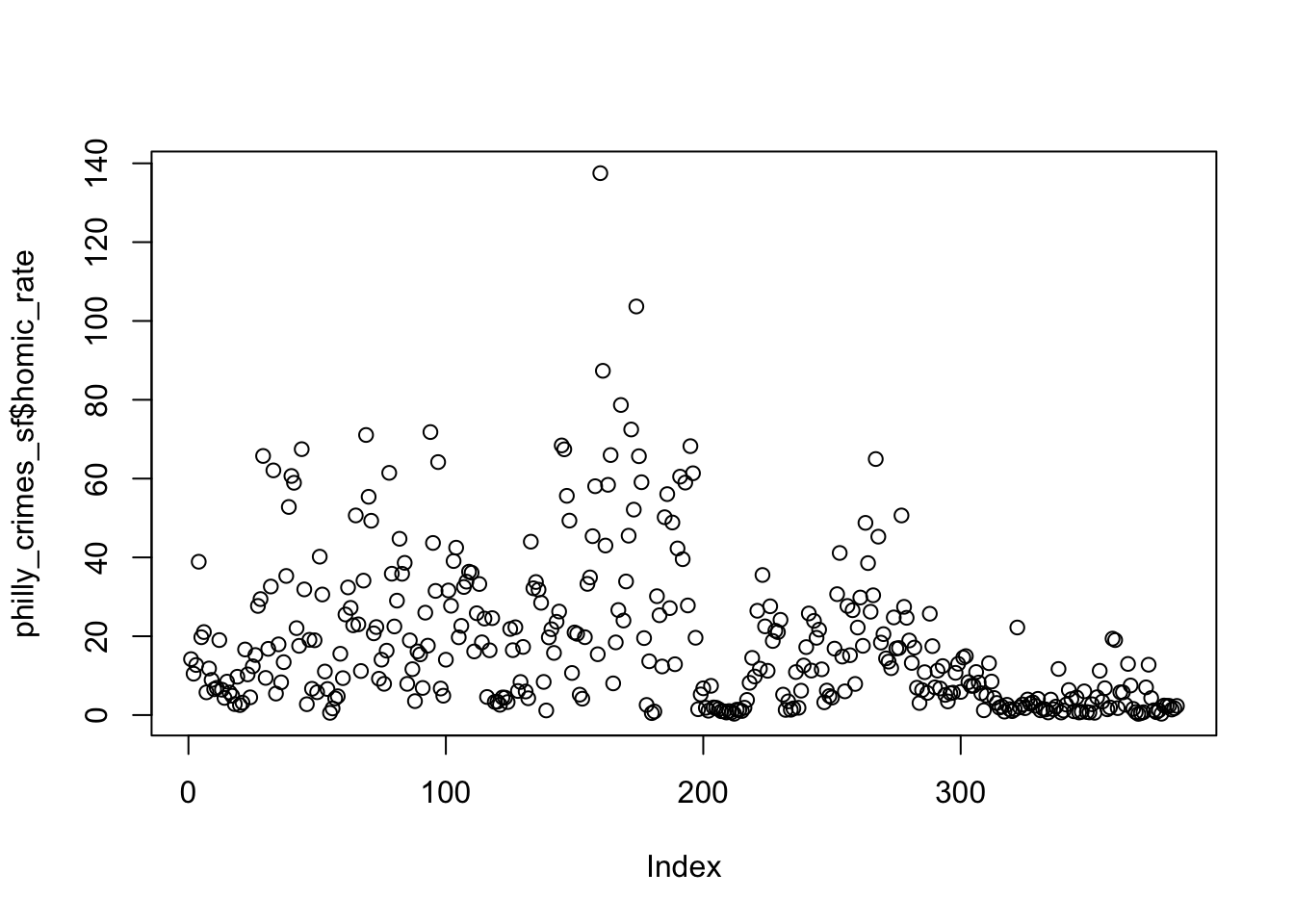``plot(philly_crimes_sf["homic_rate"])``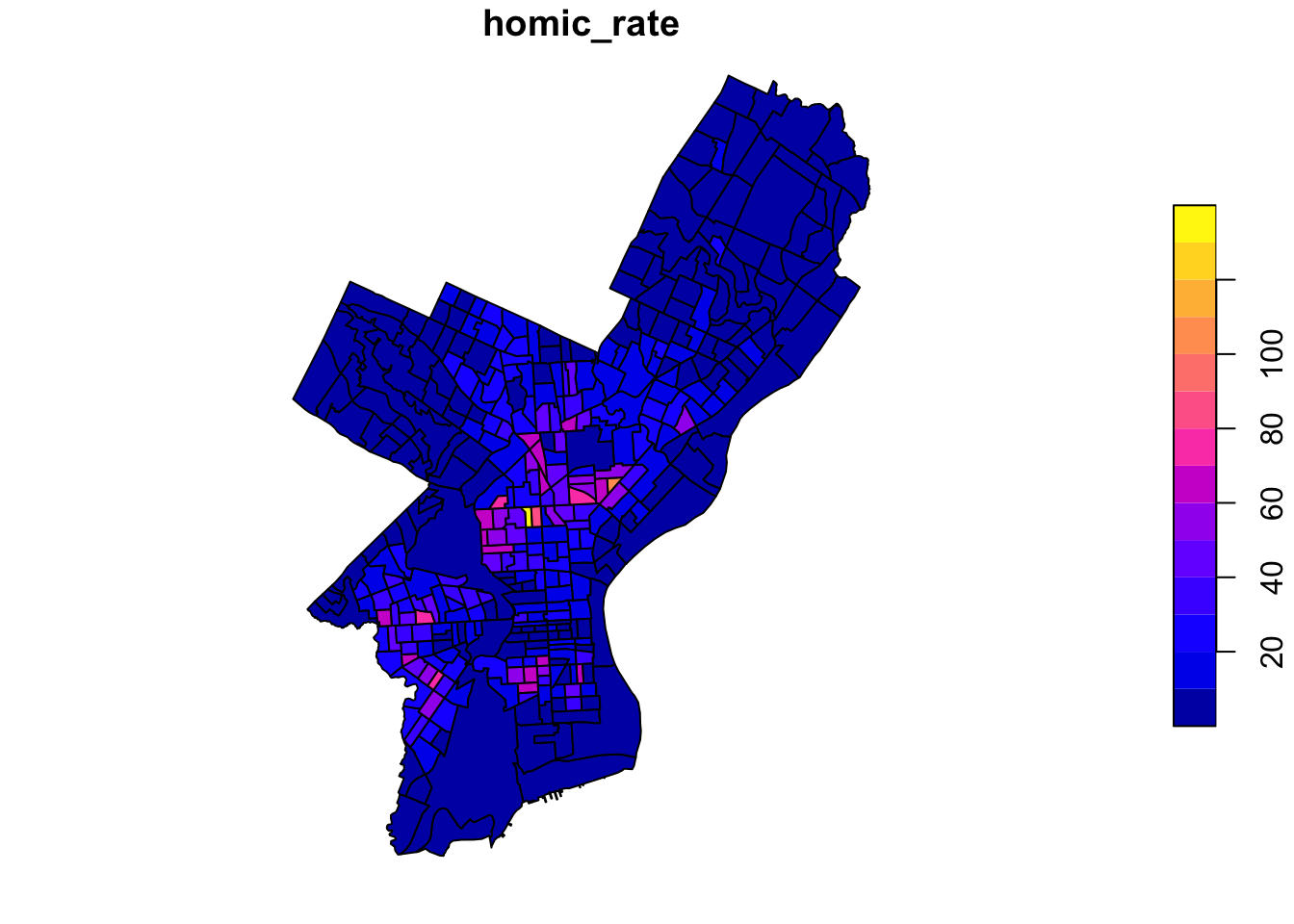Since our values are unevenly distributed…: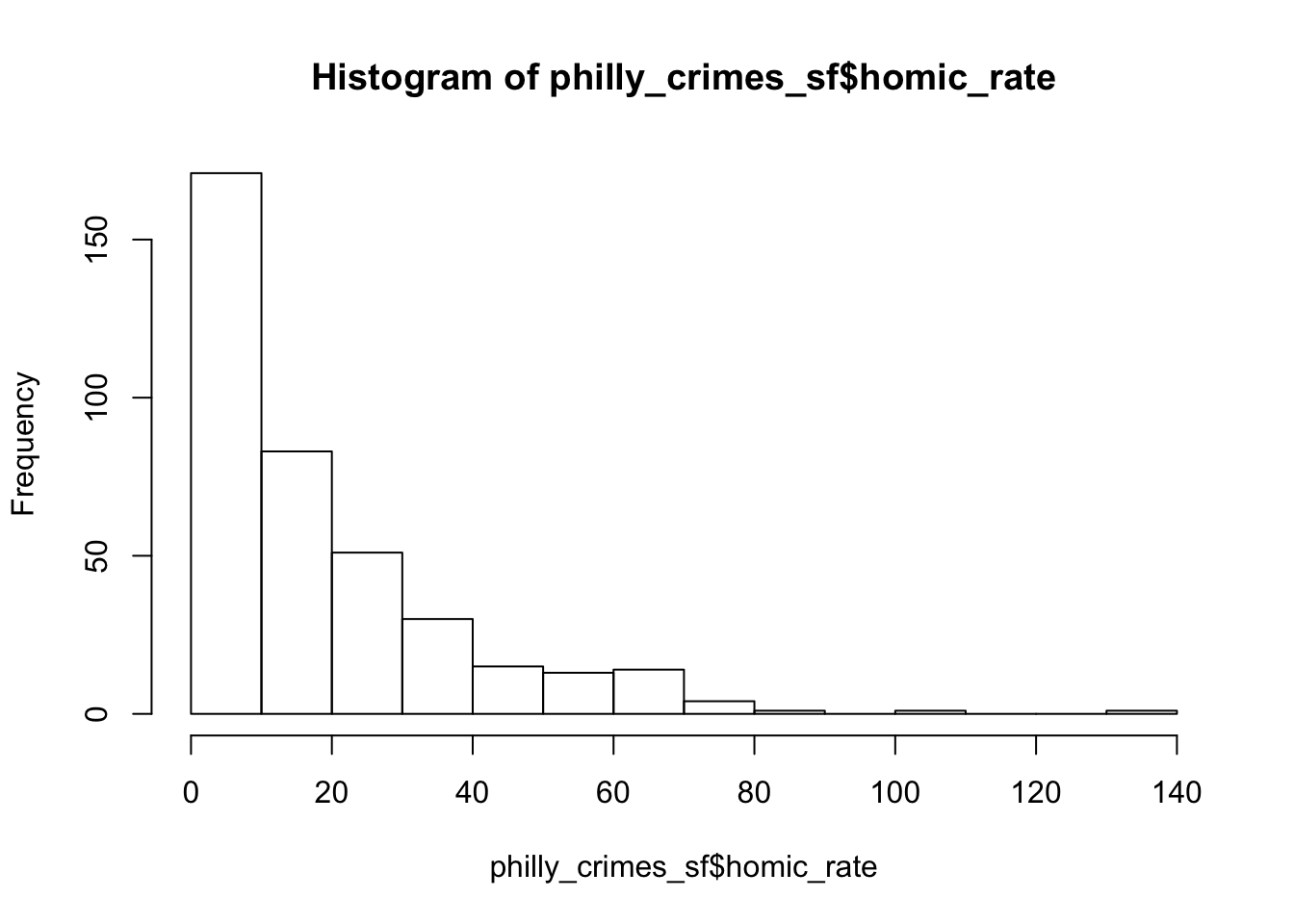…we might want to set the breaks to quantiles in order to better distinguish the census tracts with low values. This can be done by using the `breaks` argument for the sf plot function.

``````plot(philly_crimes_sf["homic_rate"],
main = "Philadelphia homicide density per square km",
breaks = "quantile")``````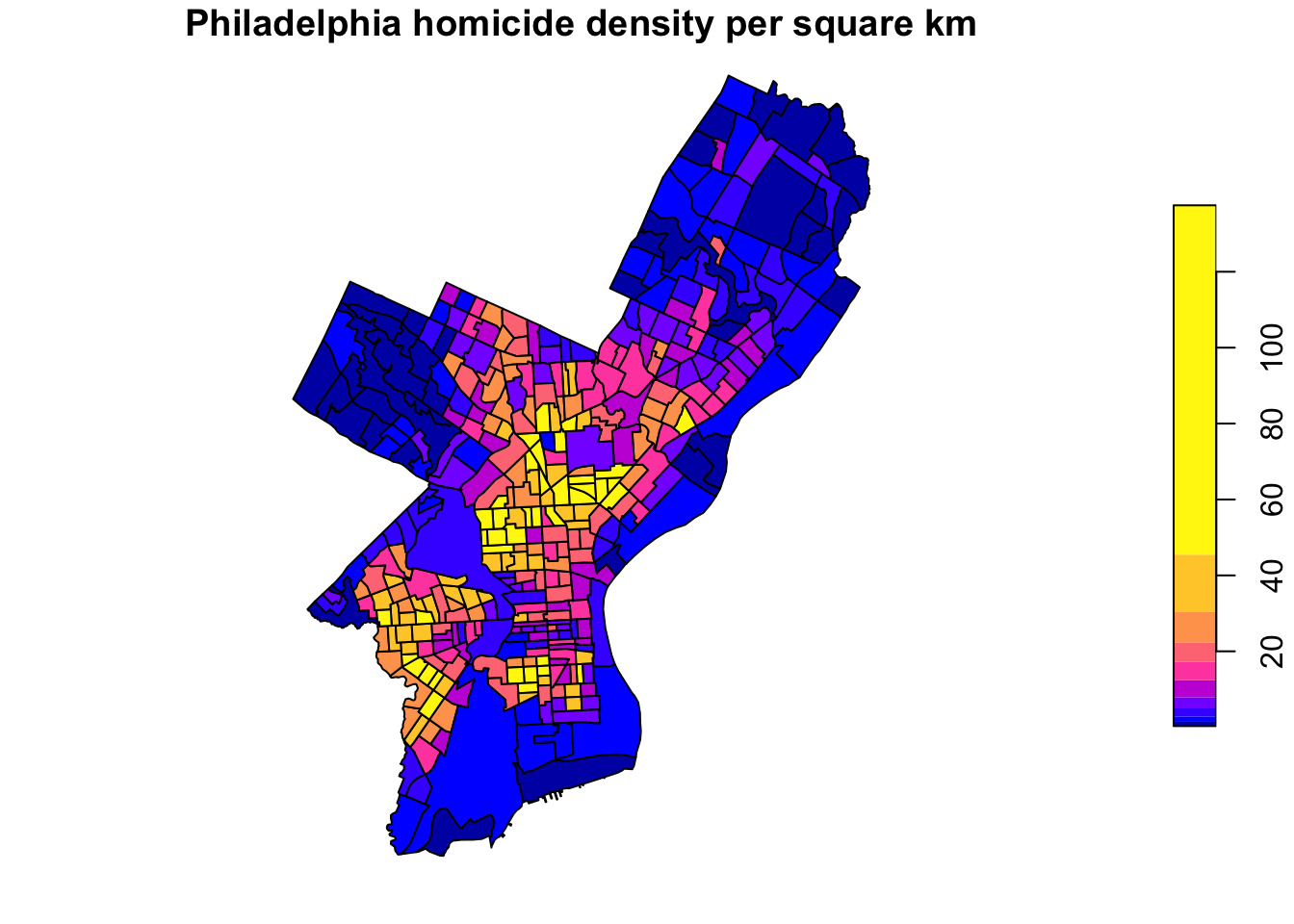We can change the color palette using a library called `RColorBrewer`11. For more about ColorBrewer palettes read this.

To make the color palettes from ColorBrewer available as R palettes we use the `brewer.pal()` function. It takes two arguments: - the number of different colors desired and - the name of the palette as character string.

We select 7 colors from the ‘Orange-Red’ plaette and assign it to an object `pal`.

``````library(RColorBrewer)
pal <- brewer.pal(7, "OrRd") # we select 7 colors from the palette
class(pal)``````
``#>  "character"``

Finally, we add this to the plot

``````plot(philly_crimes_sf["homic_rate"],
main = "Philadelphia homicide density per square km",
breaks = "quantile", nbreaks = 7,
pal = pal)``````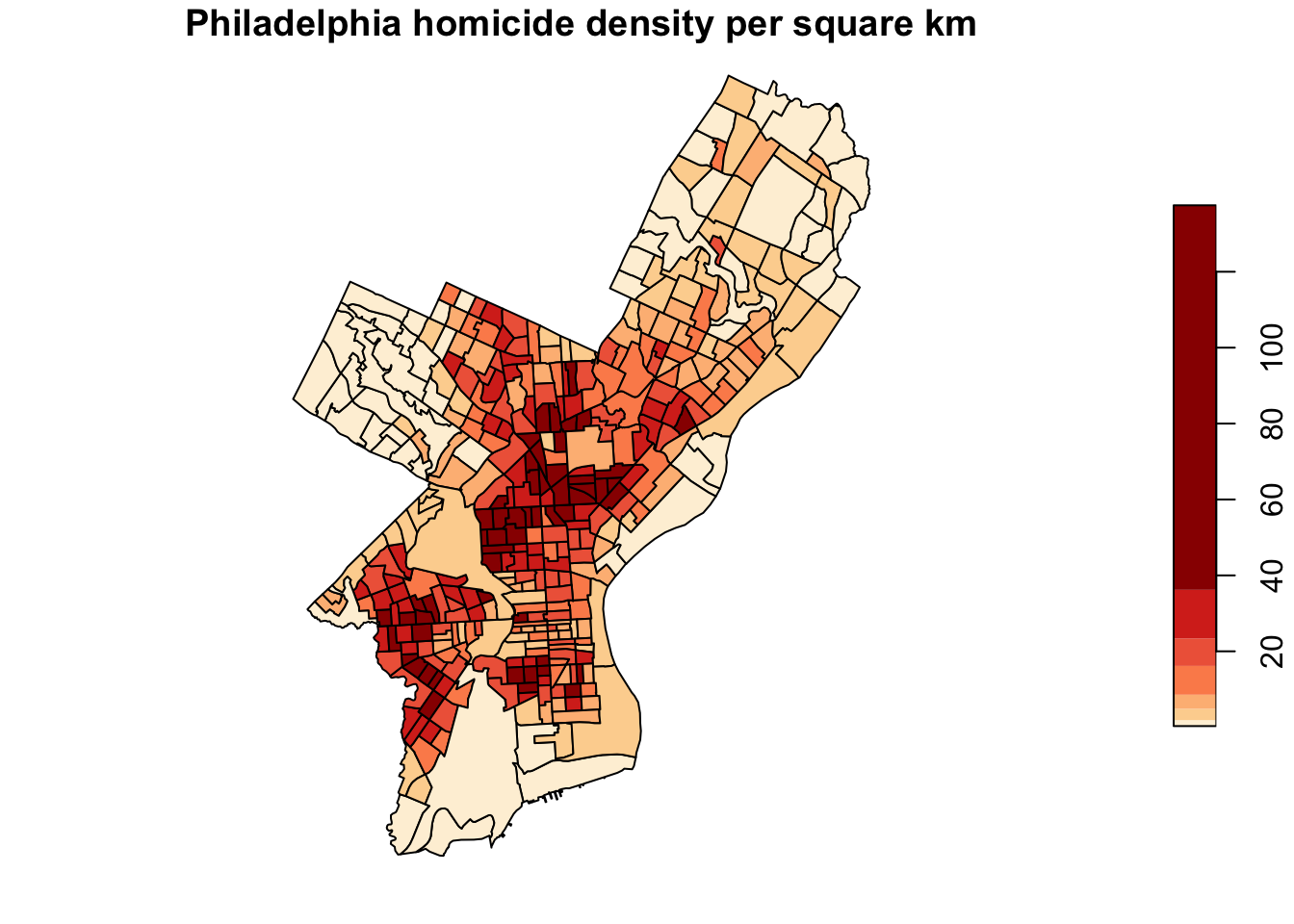## 3.2 Choropleth mapping with `spplot`

`sp` comes with a plot command `spplot()`, which takes `Spatial*` objects to plot. `spplot()` is one of the earlier functions around to plot geographic objects.

``````philly_crimes_sp <- readOGR("data/PhillyCrimerate/", "PhillyCrimerate", verbose = FALSE) # verbose = FALSE omits the message on loading
names(philly_crimes_sp)``````
``#>  "GEOID10"    "n_homic"    "tract_area" "homic_rate"``

Like plot, by default `spplot` maps all everything it can find in the attribute table. Sometimes this does not work, depending on the data types in the attribute table. In order to select specific values to map we can provide the `spplot` function with the name (or names) of the attribute variable(s) we want to plot. It is the name of the column of the `Spatial*Dataframe` as character string (or a vector if several).

``spplot(philly_crimes_sp, "homic_rate")``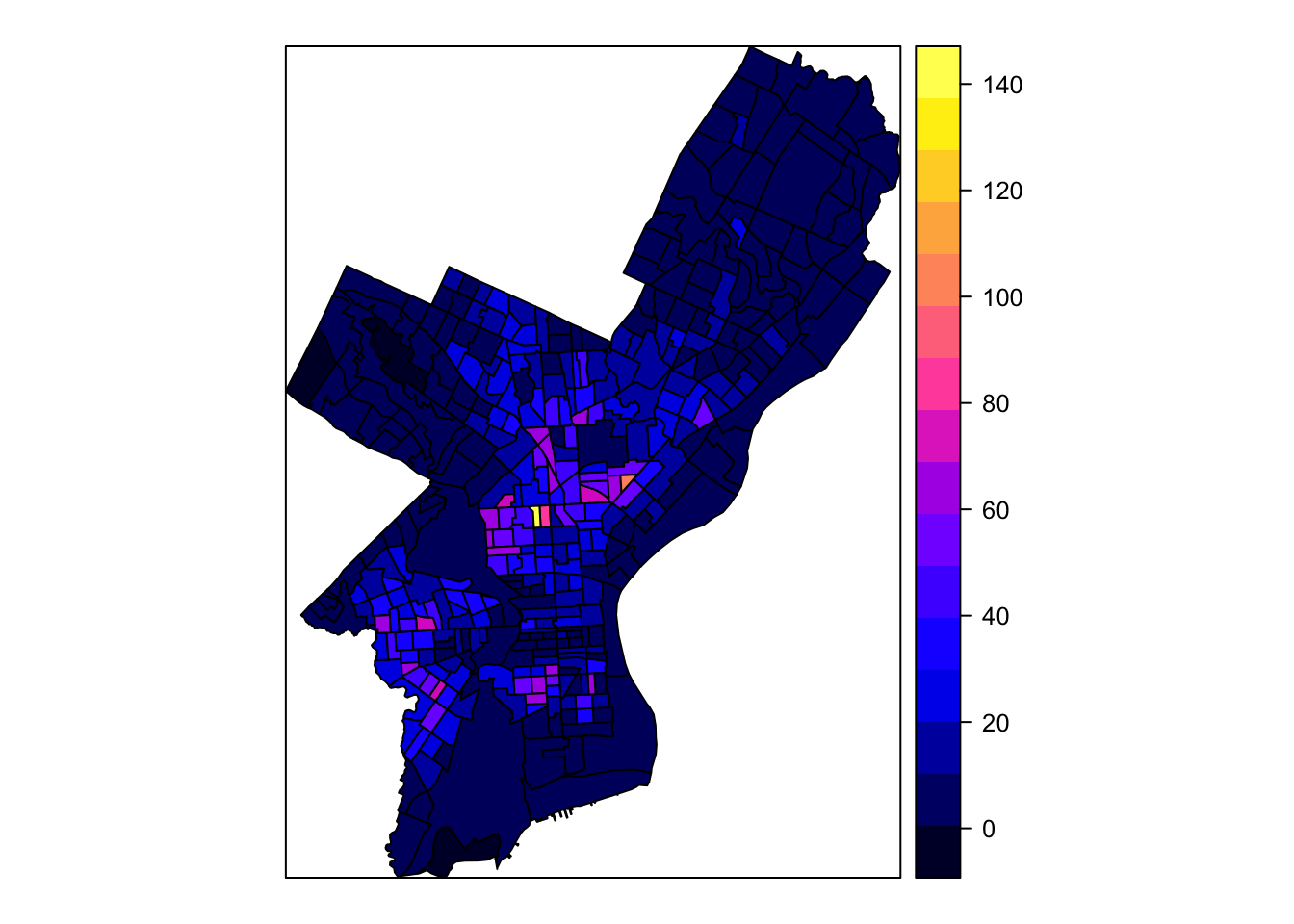Many improvements can be made here as well, below is an example12.13

``````# quantile breaks
breaks_qt <- classIntervals(philly_crimes_sp\$homic_rate, n = 7, style = "quantile")
br <- breaks_qt\$brks
offs <- 0.0000001
br <- br - offs
br[length(br)] <- br[length(br)] + offs
# categoreis for choropleth map
philly_crimes_sp\$homic_rate_bracket <- cut(philly_crimes_sp\$homic_rate, br)
# plot
spplot(philly_crimes_sp, "homic_rate_bracket", col.regions=pal, main = "Philadelphia homicide density per square km")``````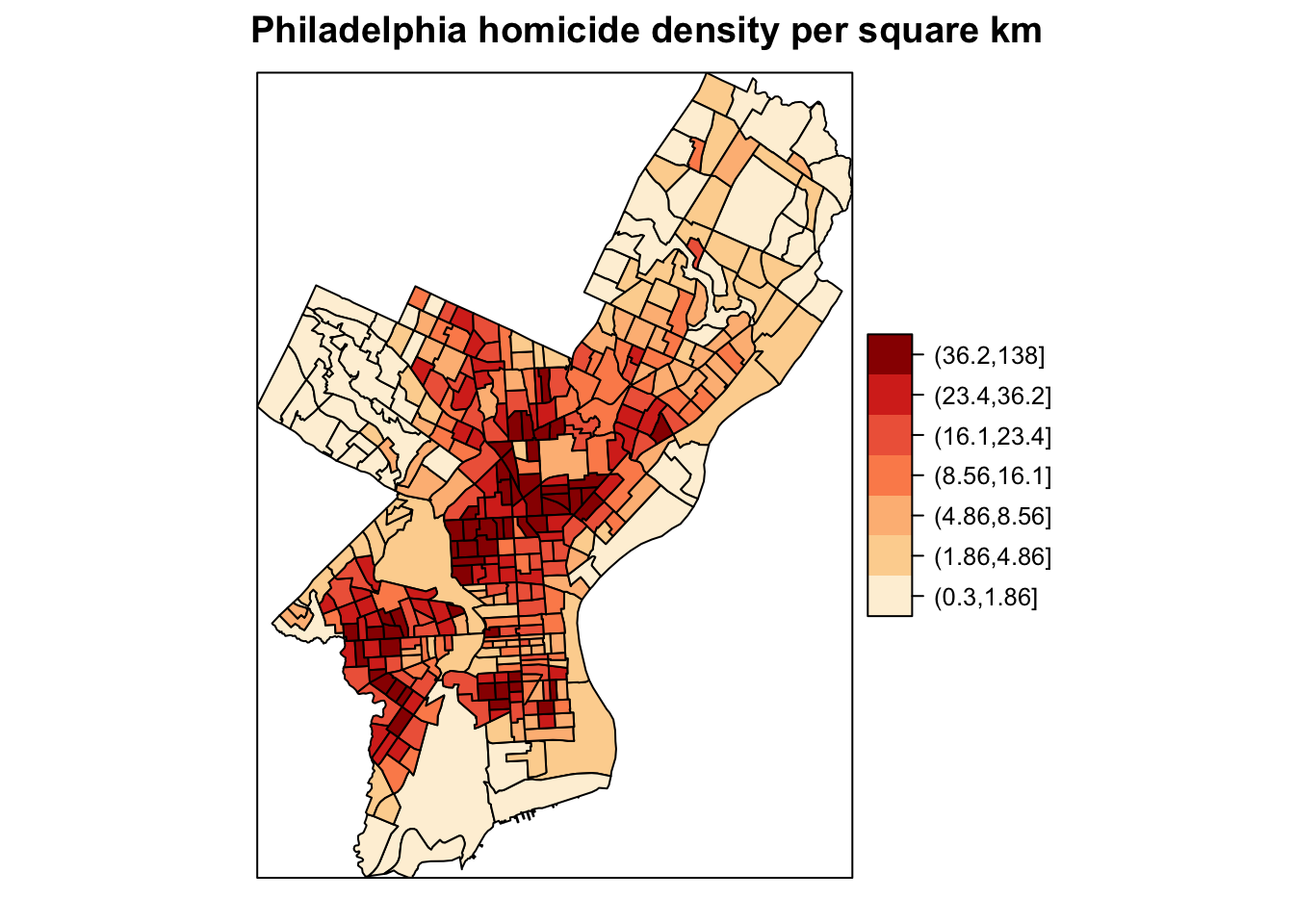## 3.3 Choropleth mapping with `ggplot2`

`ggplot2` is a widely used and powerful plotting library for R. It is not specifically geared towards mapping, but one can generate great maps.

The `ggplot()` syntax is different from the previous as a plot is built up by adding components with a `+`. You can start with a layer showing the raw data then add layers of annotations and statistical summaries. This allows to easily superimpose either different visualizations of one dataset (e.g. a scatterplot and a fitted line) or different datasets (like different layers of the same geographical area)14.

For an introduction to `ggplot` check out this book by the package creator or this for more pointers.

In order to build a plot you start with initializing a ggplot object. In order to do that `ggplot()` takes:

• a data argument usually a dataframe and
• a mapping argument where x and y values to be plotted are supplied.

In addition, minimally a geometry to be used to determine how the values should be displayed. This is to be added after an `+`.

``````ggplot(data = my_data_frame, mapping = aes(x = name_of_column_with_x_value, y = name_of_column_with_y_value)) +
geom_point()``````

Or shorter:

``````ggplot(my_data_frame, aes(name_of_column_with_x_value, name_of_column_with_y_value)) +
geom_point()``````

The great news is that `ggplot` can plot `sf` objects directly by using `geom_sf`. So all we have to do is:

``````ggplot(philly_crimes_sf) +
geom_sf(aes(fill=homic_rate))``````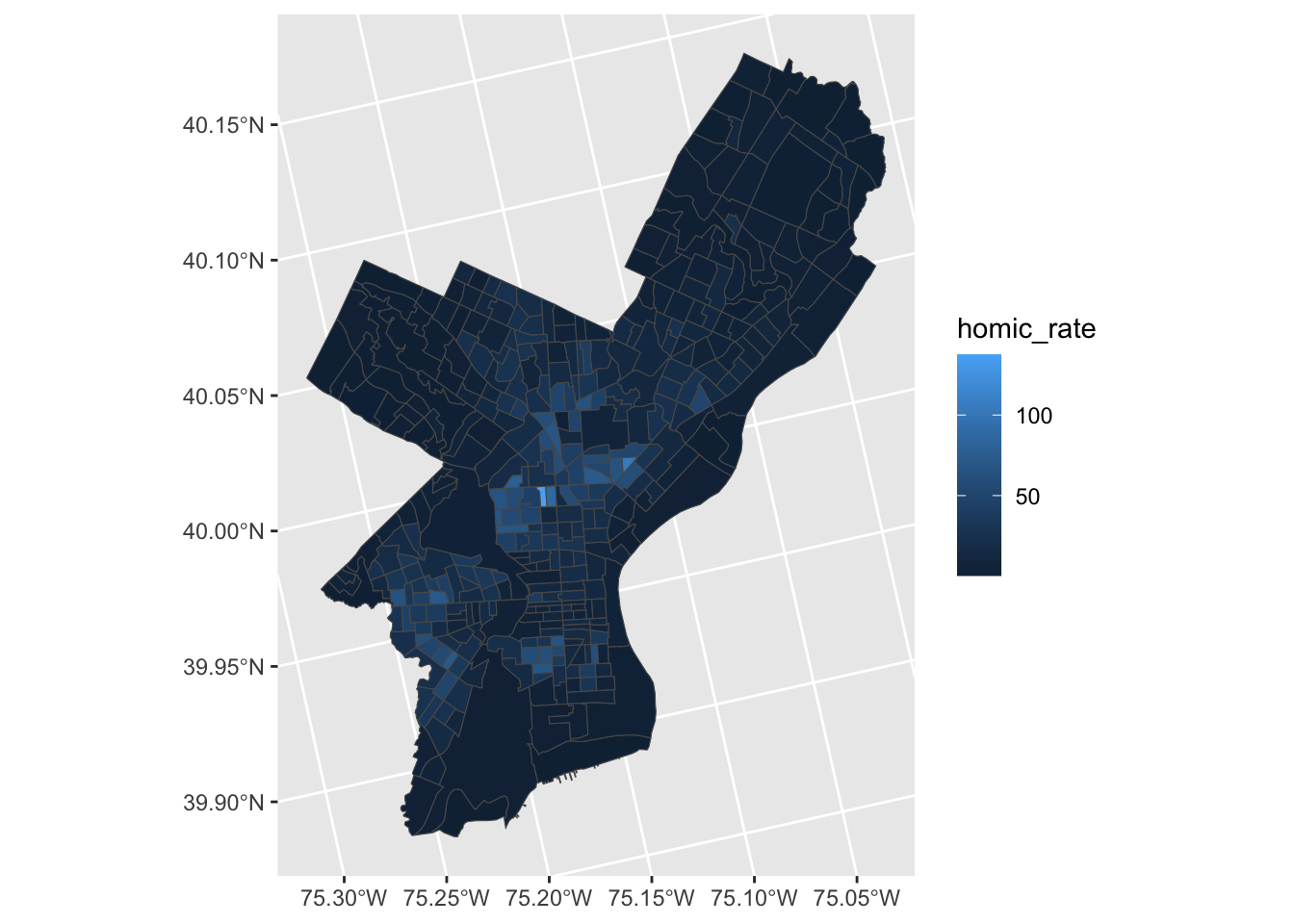Homicide rate is a continuous variable and is plotted by `ggplot` as such. If we wanted to plot our map as a ‘true’ choropleth map we need to convert our continouse variable into a categoriacal one, according to whichever brackets we want to use.

This requires two steps:

• Determine the quantile breaks.
• Add a categorical variable to the object which assigns each continious vaule to a bracket.

We will use the `classInt` package to explicitly determine the breaks.

``````library(classInt)

# get quantile breaks. Add .00001 offset to catch the lowest value
breaks_qt <- classIntervals(c(min(philly_crimes_sf\$homic_rate) - .00001, philly_crimes_sf\$homic_rate), n = 7, style = "quantile")

breaks_qt``````
``````#> style: quantile
#> [0.2997339,1.856692)  [1.856692,4.814503)  [4.814503,8.495498)
#>                   55                   55                   55
#>  [8.495498,16.14234)  [16.14234,23.20642)  [23.20642,36.16089)
#>                   55                   55                   55
#>  [36.16089,137.5028]
#>                   55``````

Ok. We can retrieve the breaks with `breaks\$brks`.

We use `cut` to divice `homic_rate` into intervals and code them according to which interval they are in.

Lastly, we can use `scale_fill_brewer` and add our color palette.

``````philly_crimes_sf <- mutate(philly_crimes_sf, homic_rate_cat = cut(homic_rate, breaks_qt\$brks))

ggplot(philly_crimes_sf) +
geom_sf(aes(fill=homic_rate_cat)) +
scale_fill_brewer(palette = "OrRd") ``````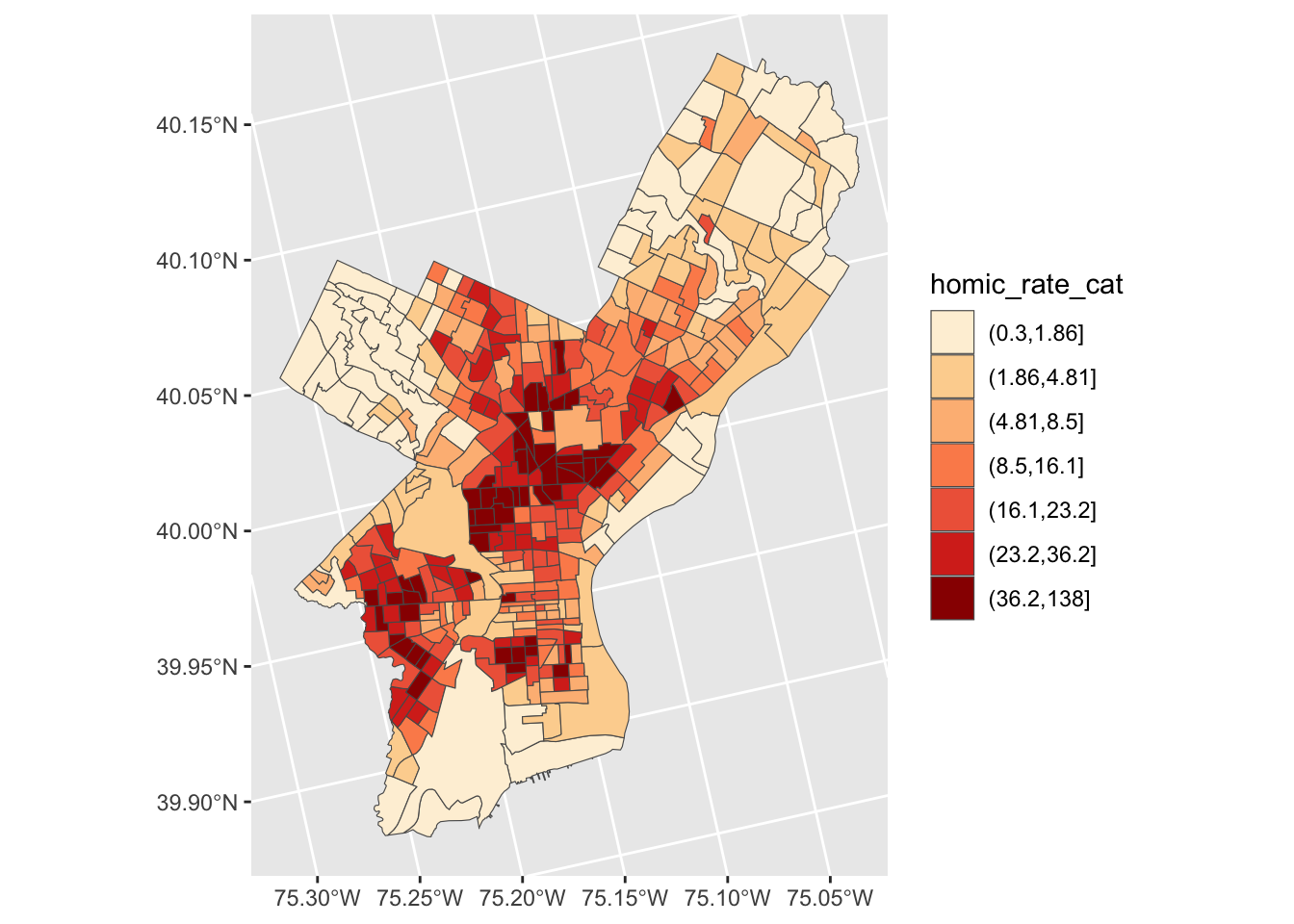## 3.4 Adding basemaps with `ggmap`

`ggmap` builds on `ggplot` and allows to pull in tiled basemaps from different services, like Google Maps, OpenStreetMaps, or Stamen Maps15.

So let’s overlay the map from above on a terrain map we pull from Stamen.

First we use the `get_map()` command from `ggmap` to pull down the basemap. We need to tell it the location or the boundaries of the map, the zoom level, and what kind of map service we like (default is Google terrain). It will actually download the tile. `get_map()` returns a ggmap object, name it `ph_basemap`. In order to view the map we then use `ggmap()`.

``````library(ggmap)

# Philadelphia Lat 39.95258 and Lon is -75.16522
ph_basemap <- get_map(location=c(lon = -75.16522, lat = 39.95258), zoom=11, maptype = 'terrain-background', source = 'stamen')

ggmap(ph_basemap)``````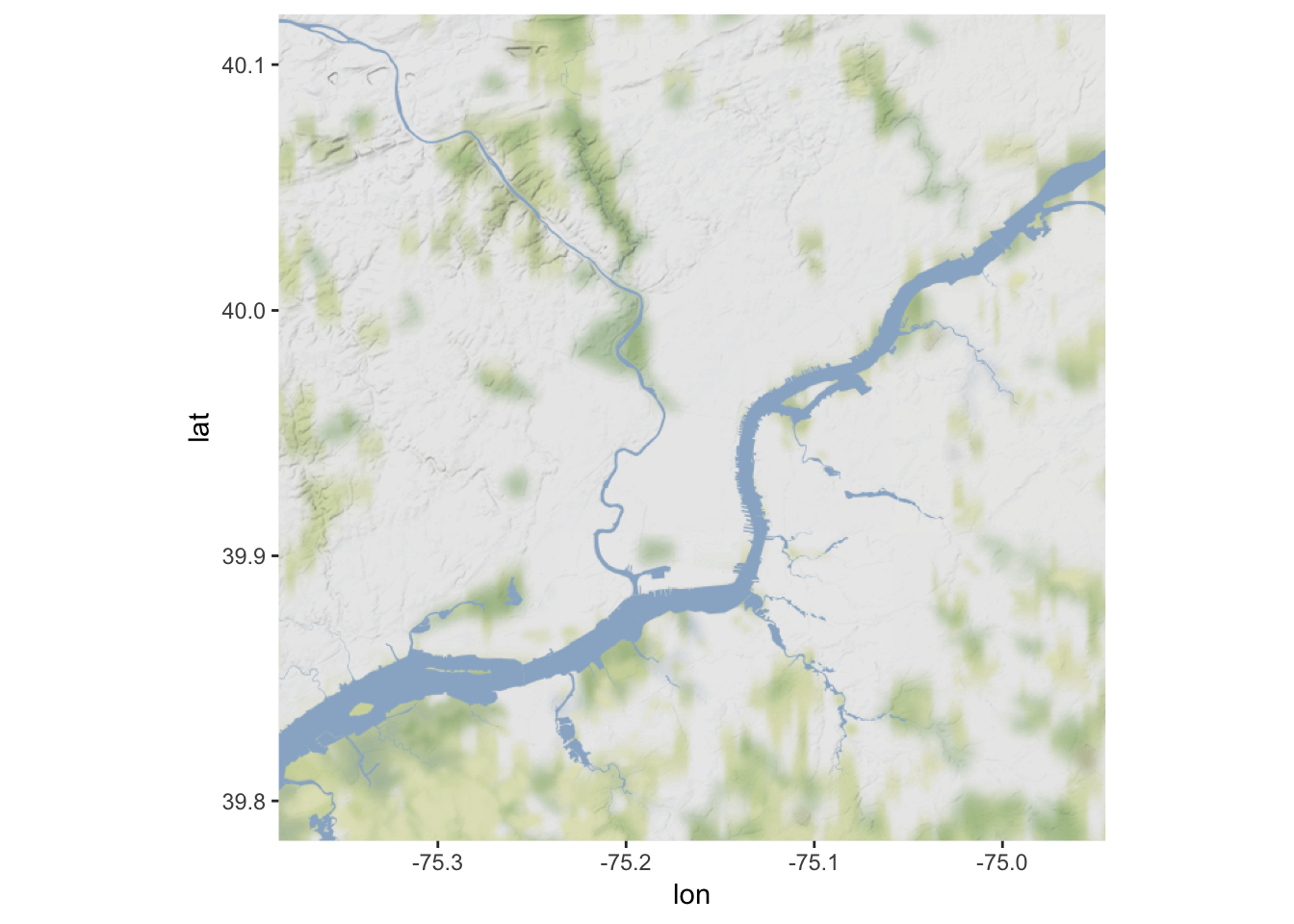Then we can reuse the code from the ggplot example above, just replacing the first line, where we initialized a ggplot object above

``    ggplot() + ``

with the line to call our basemap:

``    ggmap(ph_basemap) +``

We also have to set `inherit.aes` to `FALSE`, so it overrides the default aesthetics (from the ggmap object).

``````ggmap(ph_basemap) +
geom_sf(data = philly_crimes_sf, aes(fill=homic_rate_cat), inherit.aes = FALSE) +
scale_fill_brewer(palette = "OrRd")``````
``#> Coordinate system already present. Adding new coordinate system, which will replace the existing one.``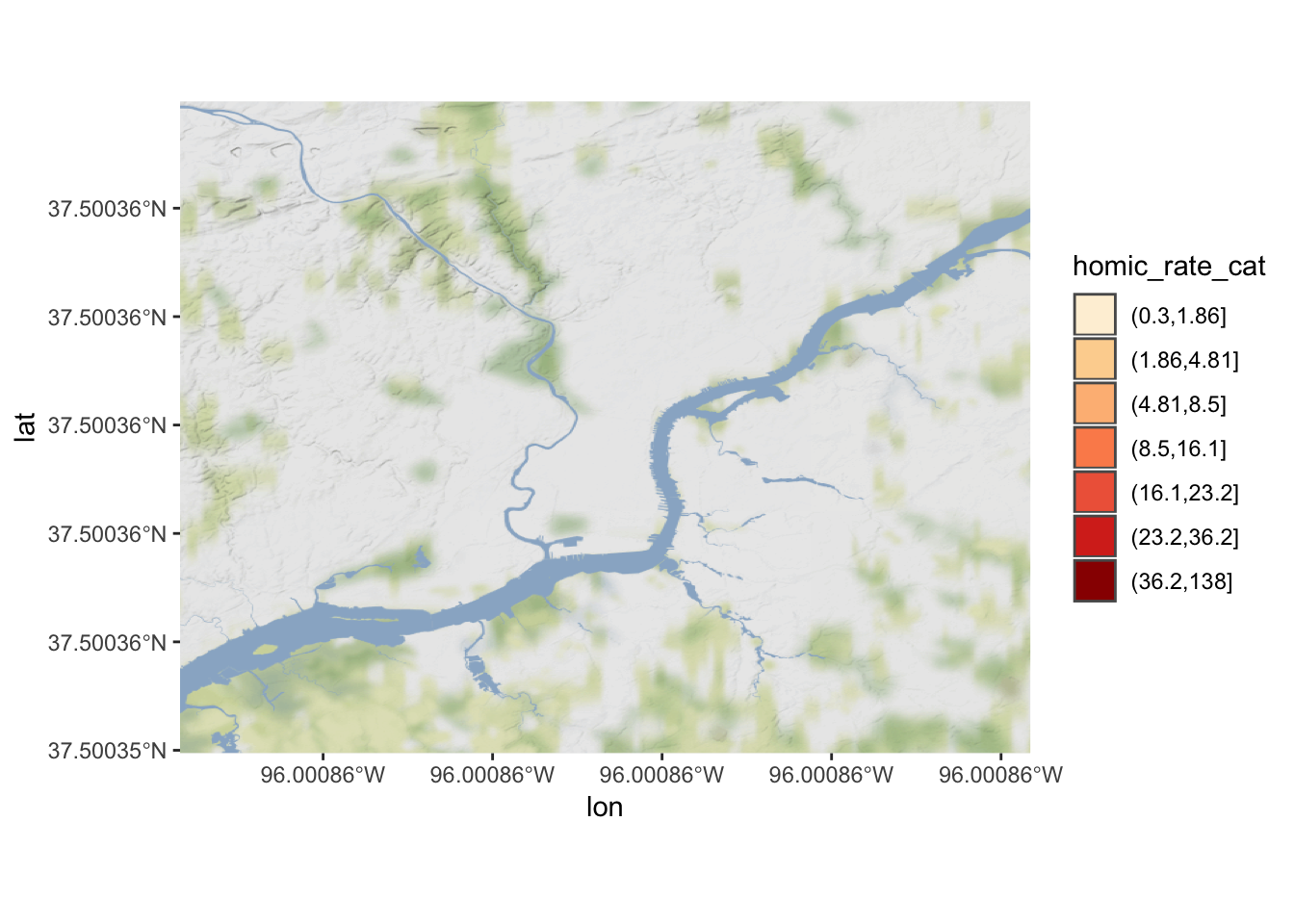Oops. Any idea what might be going on?

We need to set our CRS to WGS84, which is the one the tiles are downloaded in. We can add `coord_sf` to do this:

``````ggmap(ph_basemap) +
geom_sf(data = philly_crimes_sf, aes(fill=homic_rate_cat), inherit.aes = FALSE) +
scale_fill_brewer(palette = "OrRd") +
coord_sf(crs = st_crs(4326))``````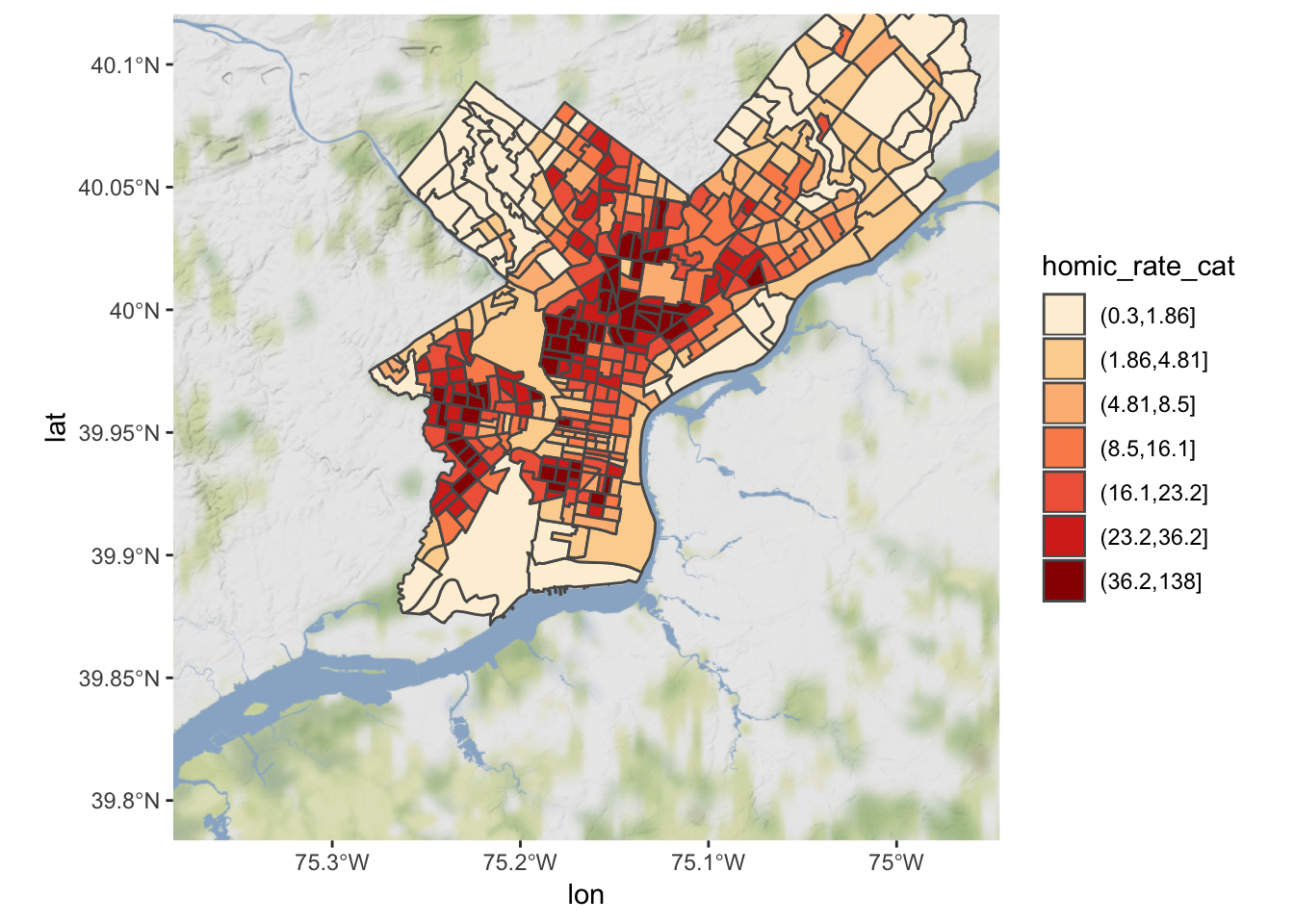The `ggmap` package also includes functions for distance calculations, geocoding, and calculating routes.

## 3.5 Choropleth with `tmap`

`tmap` is specifically designed to make creation of thematic maps more convenient. It borrows from teh ggplot syntax and takes care of a lot of the styling and aesthetics. This reduces our amount of code significantly. We only need:

• `tm_shape()` where we provide
• the `sf` object (we could also provide an `SpatialPolygonsDataframe`)
• `tm_polygons()` where we set
• the attribute variable to map,
• the break style, and
• a title.
``````library(tmap)
tm_shape(philly_crimes_sf) +
tm_polygons("homic_rate",
style="quantile",
title="Philadelphia \nhomicide density \nper sqKm")``````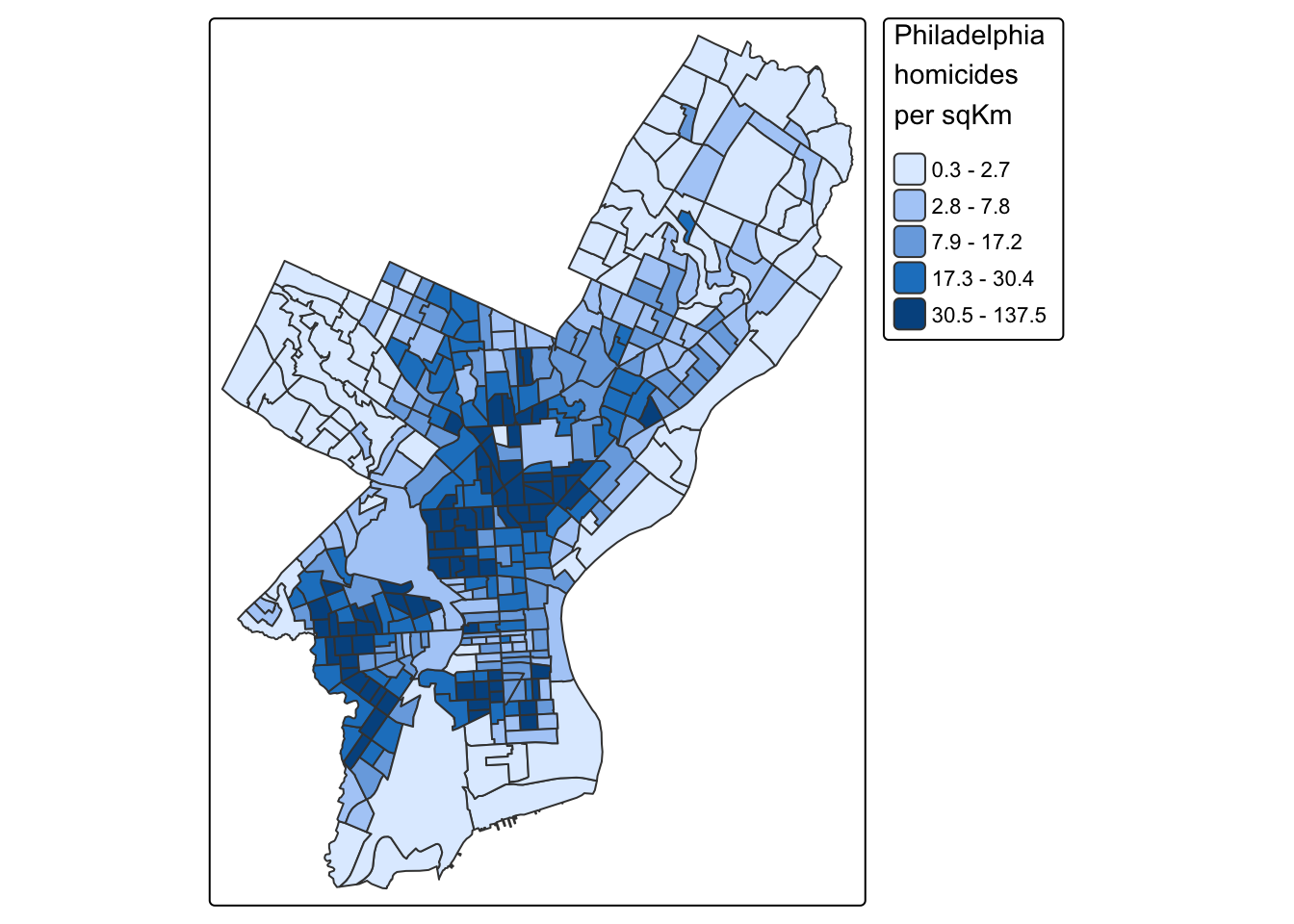`tmap` has a very nice feature that allows us to give basic interactivity to the map. We can switch from “plot” mode into “view” mode and call the last plot, like so:

``````tmap_mode("view")
tmap_last()``````

Cool huh?

The `tmap` library also includes functions for simple spatial operations, geocoding and reverse geocoding using OSM. For more check `vignette("tmap-getstarted")`.

## 3.6 Web mapping with `leaflet`

`leaflet` provides bindings to the ‘Leaflet’ JavaScript library, “the leading open-source JavaScript library for mobile-friendly interactive maps”. We have already seen a simple use of leaflet in the `tmap` example.

The good news is that the `leaflet` library gives us loads of options to customize the web look and feel of the map.

The bad news is that the `leaflet` library gives us loads of options to customize the web look and feel of the map.

Let’s build up the map step by step.

First we load the `leaflet` library. Use the `leaflet()` function with an `sp` or `Spatial*` object and pipe it to `addPolygons()` function. It is not required, but improves readability if you use the pipe operator `%>%` to chain the elements together when building up a map with `leaflet`.

And while `tmap` was tolerant about our AEA projection of `philly_crimes_sf`, `leaflet` does require us to explicitly reproject the `sf` object.

``````library(leaflet)

# reproject
philly_WGS84 <- st_transform(philly_crimes_sf, 4326)

leaflet(philly_WGS84) %>%
addPolygons()``````

To map the homicide density we use `addPolygons()` and:

• remove stroke (polygon borders)
• set a fillColor for each polygon based on `homic_rate` and make it look nice by adjusting fillOpacity and smoothFactor (how much to simplify the polyline on each zoom level). The fill color is generated using `leaflet`’s `colorQuantile()` function, which takes the color scheme and the desired number of classes. To constuct the color scheme `colorQuantile()` returns a function that we supply to `addPolygons()` together with the name of the attribute variable to map.
• add a popup with the `homic_rate` values. We will create as a vector of strings, that we then supply to `addPolygons()`.
``````pal_fun <- colorQuantile("YlOrRd", NULL, n = 5)

p_popup <- paste0("<strong>Homicide Density: </strong>", philly_WGS84\$homic_rate)

leaflet(philly_WGS84) %>%
addPolygons(
stroke = FALSE, # remove polygon borders
fillColor = ~pal_fun(homic_rate), # set fill color with function from above and value
fillOpacity = 0.8, smoothFactor = 0.5, # make it nicer
popup = p_popup)  # add popup``````

Here we add a basemap, which defaults to OSM, with `addTiles()`

``````leaflet(philly_WGS84) %>%
addPolygons(
stroke = FALSE,
fillColor = ~pal_fun(homic_rate),
fillOpacity = 0.8, smoothFactor = 0.5,
popup = p_popup) %>%
addTiles()``````

Lastly, we add a legend. We will provide the `addLegend()` function with:

• the location of the legend on the map
• the function that creates the color palette
• the value we want the palette function to use
• a title
``````leaflet(philly_WGS84) %>%
addPolygons(
stroke = FALSE,
fillColor = ~pal_fun(homic_rate),
fillOpacity = 0.8, smoothFactor = 0.5,
popup = p_popup) %>%
addTiles() %>%
addLegend("bottomright",  # location
pal=pal_fun,    # palette function
values=~homic_rate,  # value to be passed to palette function
title = 'Philadelphia homicide density per sqkm') # legend title``````